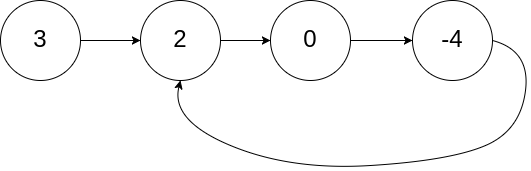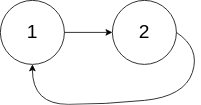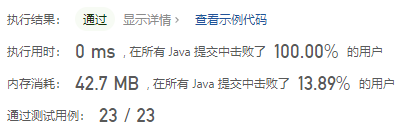#### 141. 环形链表

###### 来源: 每日一题 2023.07.29``````输入：head = [3,2,0,-4], pos = 1

````````````输入：head = [1,2], pos = 0

````````````输入：head = , pos = -1

``````

• `链表中节点的数目范围是 [0, 104]`
• `-105 <= Node.val <= 105`
• `pos 为 -1 或者链表中的一个 有效索引 。`
``````/**
* public class ListNode {
*     int val;
*     ListNode next;
*     ListNode(int x) { val = x; }
* }
*/
class Solution {

}
}
``````

#### 分析与题解

• 快慢指针法

一个链表是否有环, 这个问题算的上我很早就做的前期题, 这个的题目解题思路就是快慢指针法.

• 定义两个指针, 一个移动速度为1.

``````firstNode = firstNode.next;
``````
• 第二个指针的移动速度为2.

``````secondNode = secondNode.next.next;
``````
• `如果链表有环, 不管转几圈, 两者必然会有一个时间点相遇`, 这就是整个题解的核心.

``````while(firstNode != null && secondNode != null) {
if(firstNode == secondNode) {
return true;
}
if (secondNode.next == null) {
secondNode = null;
} else {
secondNode = secondNode.next.next;
}
firstNode = firstNode.next;
}
``````
• 如果一个正常链表没有环,那么这两个指针移动到最后就会变成 `null` , 这也是停止循环的条件.

整个解题过程如下所示.

``````/**
* class ListNode {
*     int val;
*     ListNode next;
*     ListNode(int x) {
*         val = x;
*         next = null;
*     }
* }
*/
public class Solution {
// 判断有环就用两个快慢指针
return false;
} else {
}
while(firstNode != null && secondNode != null) {
if(firstNode == secondNode) {
return true;
}
if (secondNode.next == null) {
secondNode = null;
} else {
secondNode = secondNode.next.next;
}
firstNode = firstNode.next;
}
return false;
}
}
``````

复杂度分析:

• 时间复杂度: O(n)
• 空间复杂度: O(1)

结果如下所示.IT界无底坑洞栋主 欢迎加Q骚扰:676758285